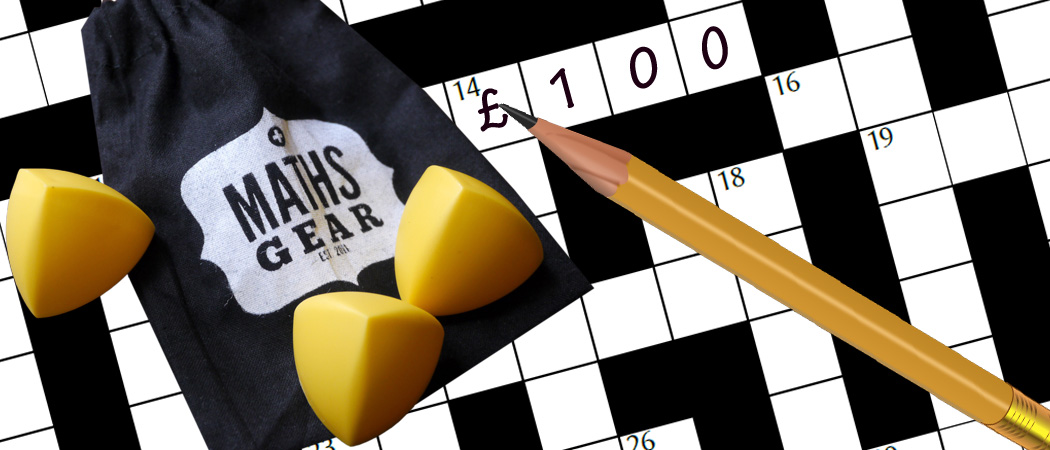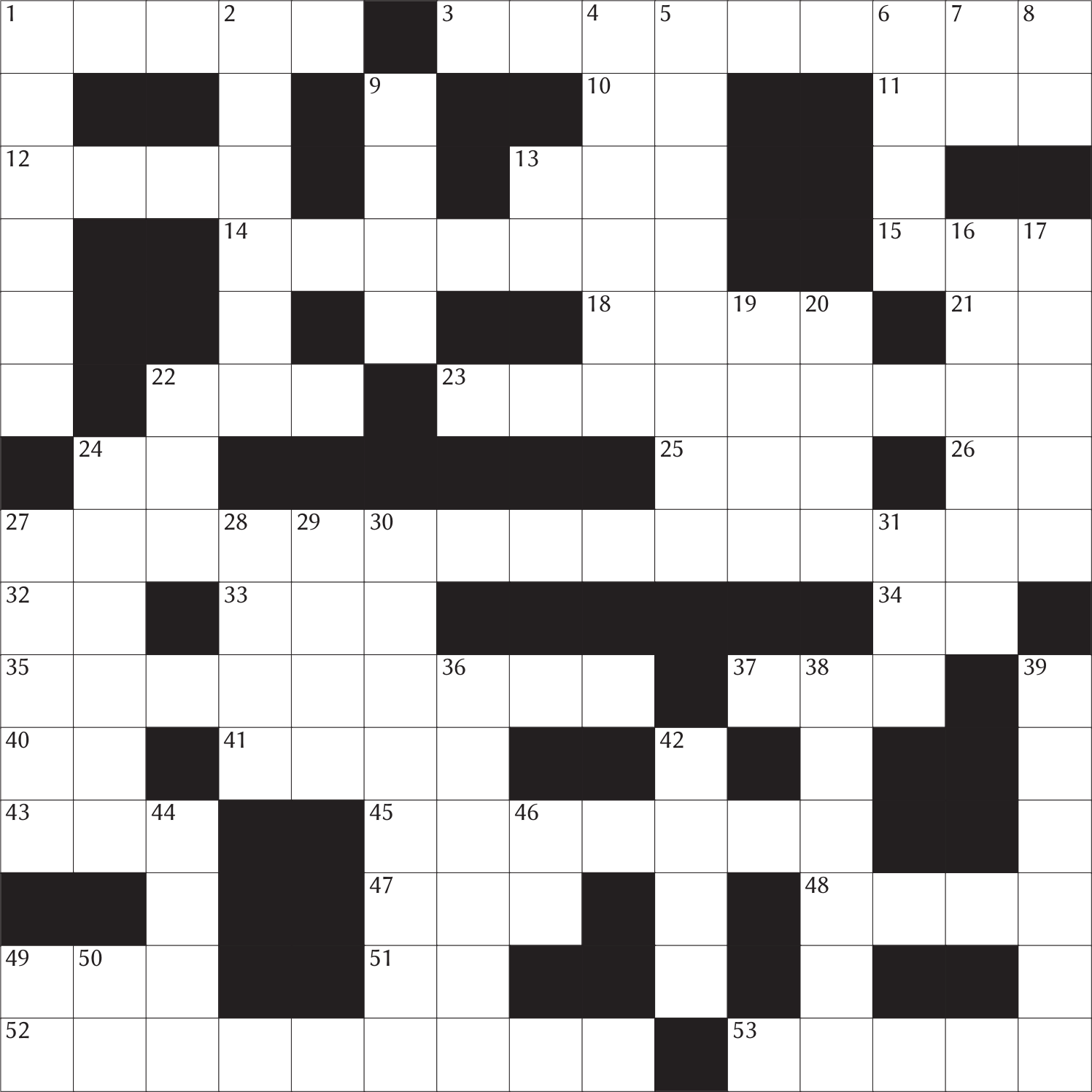# Prize crossnumber, Issue 10

Can you solve it?Our original prize crossnumber is featured on pages 48 and 49 of Issue 10.

### Rules

• Although many of the clues have multiple answers, there is only one solution to the completed crossnumber. As usual, no numbers begin with 0. Use of Python, OEIS, Wikipedia, etc. is advised for some of the clues.
• One randomly selected correct answer will win a £100 Maths Gear goody bag, including non-transitive dice, a Festival of the Spoken Nerd DVD, and much, much more. Three randomly selected runners up will win a Chalkdust T-shirt. Maths Gear is a website that sells nerdy things worldwide, with free UK shipping.
• To enter, enter the sum of the across clues below by 2 February 2020. Only one entry per person will be accepted. Winners will be notified by email and announced on our blog by 14 March 2020.

Correction: Clue 13A has been corrected to “49A reversed” instead of “49D reversed”.
Correction: Clue 38D has been corrected to “33A less than 39D” instead of “33A less than 39A”.
Clarificiation: The triangle in 6D is a right-angled triangle.

### Crossnumber

Crossnumber #10, set by Humbug:Click to enlarge.

### Clues

#### Across

• 1 9 times this number is an anagram of this number. (5)
• 3 All the digits of this number are equal. (9)
• 10 Not equal to 32A or 40A. (2)
• 11 A multiple of 8D. (3)
• 12 The sum of 25A and 43A. (4)
• 13 49A reversed. (3)
• 14 Each digit of this number (except the first) is either one less or two less than the previous digit. (7)
• 15 31D reversed. (3)
• 18 5 more than a palindrome. (4)
• 21 The highest common factor of 26A and 34A. (2)
• 22 6 more than a palindrome. (3)
• 23 Each digit of this number (except the first) is either one more or three less than the previous digit. (9)
• 24 Not equal to 32A or 40A. (2)
• 25 The difference between 31D and 15A. (3)
• 26 The highest common factor of 21A and 34A. (2)
• 27 Each digit of this number (except the first and last) is either the sum or the difference of the digits either side of it. (15)
• 32 The highest common factor of 10A and 24A. (2)
• 33 3 more than a palindrome. (3)
• 34 The highest common factor of 21A and 26A. (2)
• 35 Each digit of this number (except the first) is either one more or three less than the previous digit. (9)
• 37 The difference between 49A and 13A. (3)
• 40 The lowest common multiple of 10A and 24A. (2)
• 41 4 more than a palindrome. (4)
• 43 25A reversed. (3)
• 45 7 more than a palindrome. (7)
• 47 37A reversed. (3)
• 48 The sum of 47A and 37A. (4)
• 49 Each digit of this number (except the first) is (strictly) less than the previous digit. (3)
• 51 The sum of the digits of 23A. (2)
• 52 All the digits of this number are equal. (9)
• 53 Equal to 1A. (5)

#### Down

• 1 A multiple of 9D. (6)
• 2 A multiple of 1D. (6)
• 4 One of the digits of 33A appears more than once in this number. (6)
• 5 The sum of this number, 23A and 30D is greater than 1,000,000,000. (8)
• 6 The length of one of the other two sides of a triangle whose hypotenuse is 2665 units long and whose sides all have integer length. (4)
• 7 A solution of $x^2-(\text{50D})x+(\text{11A})=0$. (2)
• 8 A solution of $x^2-(\text{50D})x+(\text{11A})=0$. (2)
• 9 A palindrome. (4)
• 13 A prime number. (2)
• 16 Each digit of this number (except the first and last) is either the sum or the difference of the digits either side of it. (6)
• 17 The sum of this number’s digits is the square of its first digit. (5)
• 19 2 more than a palindrome. (4)
• 20 The sum of this number and 33A is greater than 10,000. (4)
• 22 A non-prime number with only one (distinct) prime factor. (3)
• 24 This number contains only two different digits. (6)
• 27 A multiple of 13D. (5)
• 28 Each digit of this number (except the first) is one less than the previous digit. (4)
• 29 8 more than a palindrome. (4)
• 30 A multiple of the final digit of 22A. (8)
• 31 The sum of this number’s digits is 7. (3)
• 36 A multiple of 9 that is less than 4D. (6)
• 38 33A less than 39D. (6)
• 39 Equal to 2D. (6)
• 42 Equal to 18A. (4)
• 44 1 more than a palindrome. (4)
• 46 A factor of 14A. (2)
• 49 The first digit of this number is one of the digits of 45A. (2)
• 50 More than 8D. (2)